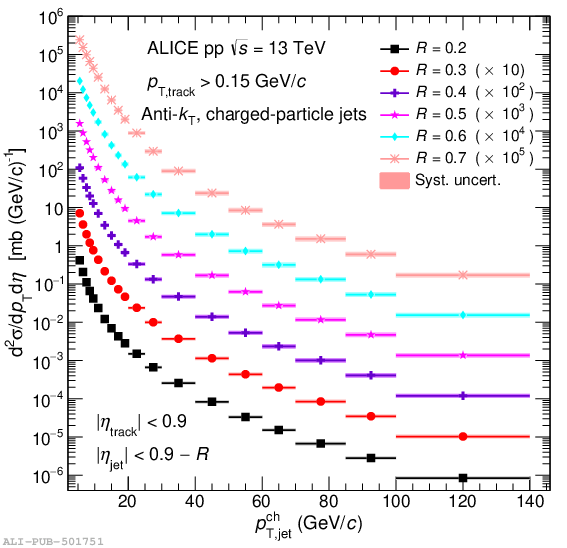# Figure 15

 Inclusive charged-particle jet cross sections in pp collisions at $\sqrt{s} =$ 13 TeV using the anti-$k_{\rm T}$ algorithm for different resolution parameters $R$ varied from $0.2$ to $0.7$, without UE subtraction. Statistical uncertainties are displayed as vertical error bars. The total systematic uncertainties are shown as solid boxes around the data points.